卷积神经网络应用：基于Tensorflow的CNN/CRF图像分割技术

+关注继续查看

上采样辅助函数与图像加载

import numpy as np

def get_kernel_size(factor):
"""
给定所需的上采样因子，确定卷积核的大小
"""
return 2 * factor - factor % 2

def upsample_filt(size):
"""
创建一个给定(h, w) 大小的适用于上采样过程的二维双线性卷积核
"""
factor = (size + 1) // 2
if size % 2 == 1:
center = factor - 1
else:
center = factor - 0.5
og = np.ogrid[:size, :size]
return (1 - abs(og - center) / factor) * \
(1 - abs(og - center) / factor)

def bilinear_upsample_weights(factor, number_of_classes):
"""
使用双线性卷积核，为转置卷积创建权重矩阵
初始化
"""

filter_size = get_kernel_size(factor)

weights = np.zeros((filter_size,
filter_size,
number_of_classes,
number_of_classes), dtype=np.float32)

upsample_kernel = upsample_filt(filter_size)

for i in xrange(number_of_classes):

weights[:, :, i, i] = upsample_kernel

return weights
%matplotlib inline

from __future__ import division

import os
import sys
import tensorflow as tf
import skimage.io as io
import numpy as np

os.environ["CUDA_VISIBLE_DEVICES"] = '1'
sys.path.append("/home/dpakhom1/workspace/my_models/slim/")
checkpoints_dir = '/home/dpakhom1/checkpoints'

image_filename = 'cat.jpg'
annotation_filename = 'cat_annotation.png'

image_filename_placeholder = tf.placeholder(tf.string)
annotation_filename_placeholder = tf.placeholder(tf.string)
is_training_placeholder = tf.placeholder(tf.bool)

feed_dict_to_use = {image_filename_placeholder: image_filename,
annotation_filename_placeholder: annotation_filename,
is_training_placeholder: True}

image_tensor = tf.image.decode_jpeg(image_tensor, channels=3)
annotation_tensor = tf.image.decode_png(annotation_tensor, channels=1)

# 对于每个类别，将其设置为1而不是一个数字——我们在后续计算
# 交叉熵时会用到。有的时候，实际分割区域的掩码可以有很多值，
# 不仅仅为0和1
class_labels_tensor = tf.equal(annotation_tensor, 1)
background_labels_tensor = tf.not_equal(annotation_tensor, 1)

#将布尔值转换为浮点数——这样才能正确地计算交叉熵损失

# 调整输入数据的大小，使其与tf.softmax_cross_entropy_with_logits中
# [batch_size, num_classes]的要求保持一致

<img src="https://yqfile.alicdn.com/4a8f9e64c859fedd6c2346716e3a257f5ac383af.png" height="60" width="180" />

import numpy as np
import tensorflow as tf
import sys
import os
from matplotlib import pyplot as plt

fig_size = [15, 4]
plt.rcParams["figure.figsize"] = fig_size

import urllib2

slim = tf.contrib.slim

from nets import vgg
from preprocessing import vgg_preprocessing

# 加载像素均值以及为每个像素进行减法运算的函数
from preprocessing.vgg_preprocessing import (_mean_image_subtraction,
_R_MEAN, _G_MEAN, _B_MEAN)

upsample_factor = 32
number_of_classes = 2
log_folder = '/home/dpakhom1/tf_projects/segmentation/log_folder'

vgg_checkpoint_path = os.path.join(checkpoints_dir, 'vgg_16.ckpt')

# 在与像素均值做差前，将图像转换至float32类型
image_float = tf.to_float(image_tensor, name='ToFloat')

# 将每个像素的具体数值与像素均值做差
mean_centered_image = _mean_image_subtraction(image_float,
[_R_MEAN, _G_MEAN, _B_MEAN])

processed_images = tf.expand_dims(mean_centered_image, 0)

upsample_filter_np = bilinear_upsample_weights(upsample_factor,
number_of_classes)

upsample_filter_tensor = tf.constant(upsample_filter_np)

# 定义将要使用的模型——指定在最后一层仅使用两个类别
with slim.arg_scope(vgg.vgg_arg_scope()):

logits, end_points = vgg.vgg_16(processed_images,
num_classes=2,
is_training=is_training_placeholder,
spatial_squeeze=False,

downsampled_logits_shape = tf.shape(logits)

# 计算上采样数据的输出大小
upsampled_logits_shape = tf.pack([
downsampled_logits_shape,
downsampled_logits_shape * upsample_factor,
downsampled_logits_shape * upsample_factor,
downsampled_logits_shape
])

# 进行上采样处理
upsampled_logits = tf.nn.conv2d_transpose(logits, upsample_filter_tensor,
output_shape=upsampled_logits_shape,
strides=[1, upsample_factor, upsample_factor, 1])

# 展开预测结果，以便于我们计算每个像素的交叉熵，并获得交叉熵的总和
flat_logits = tf.reshape(tensor=upsampled_logits, shape=(-1, number_of_classes))

cross_entropies = tf.nn.softmax_cross_entropy_with_logits(logits=flat_logits,
labels=flat_labels)

cross_entropy_sum = tf.reduce_sum(cross_entropies)

# 获得每个像素的最终预测结果——请注意，在这种情况下我们并不需要
# 使用softmax，因为我们只需要得到最终的决策。如果我们还需要各
# 个类别的概率，那么我们必须应用softmax
pred = tf.argmax(upsampled_logits, dimension=3)

probabilities = tf.nn.softmax(upsampled_logits)

# 在这里我们定义了一个优化器，并添加了所有将要创建至命名

# 我们还获得了每个变量的梯度数据
# 这样，我们可以在tensorboard中可视化这些变量
# 等价于执行：

# 替换原始变量名中的一些字符
# tensorboard不支持':'符号

# 得到每一层的梯度直方图，并随后在tensorboard中可视化这些数据
tensorboard

# 在这里，我们定义了一个函数，调用时会从VGG模型检查点中读取权重数据，并加载至变量中。
# 我们从负责类别预测的最后一层中剔除了权重。我们这样做是因为我们将有不同数量的
# 类别进行预测，我们不能在初始化时使用原先的类别。

# 这里我们得到了网络中最后一层的权重变量
# 正如我们看到的，VGG最初训练的类别数量与我们实际的类别数量
# 并不相同——在我们的情况下，总共只有两类
vgg_fc8_weights = slim.get_variables_to_restore(include=['vgg_16/fc8'])

# 为模型损失添加一个summary OP——以便我们可以在tensorboard中看到它
tf.summary.scalar('cross_entropy_loss', cross_entropy_sum)

# 将所有summary OP合并至一个OP总
# 在运行程序时生成字符串
merged_summary_op = tf.summary.merge_all()

# 创建一个summary writer——用于将所有日志写入到一个特定文件中
# 这个文件后续可以由tensorboard读取
summary_string_writer = tf.summary.FileWriter(log_folder)

# 如果日志文件夹尚未存在，则创建一个新的文件夹
if not os.path.exists(log_folder):
os.makedirs(log_folder)

# 创建一个OP，对VGG模型中各权重变量进行初始化操作
vgg_checkpoint_path,
vgg_except_fc8_weights)

# 针对新的fc8层权重数据的初始化器——仅包括两类
vgg_fc8_weights_initializer = tf.variables_initializer(vgg_fc8_weights)

with tf.Session() as sess:
# 运行初始化器
sess.run(vgg_fc8_weights_initializer)
sess.run(optimization_variables_initializer)

train_image, train_annotation = sess.run([image_tensor, annotation_tensor],
feed_dict=feed_dict_to_use)

f, (ax1, ax2) = plt.subplots(1, 2, sharey=True)
ax1.imshow(train_image)
ax1.set_title('Input image')
probability_graph = ax2.imshow(np.dstack((train_annotation,)*3)*100)
ax2.set_title('Input Ground-Truth Annotation')
plt.show()

# 执行10次迭代
for i in range(10):

loss, summary_string = sess.run([cross_entropy_sum, merged_summary_op],
feed_dict=feed_dict_to_use)

sess.run(train_step, feed_dict=feed_dict_to_use)

pred_np, probabilities_np = sess.run([pred, probabilities],
feed_dict=feed_dict_to_use)

cmap = plt.get_cmap('bwr')

f, (ax1, ax2) = plt.subplots(1, 2, sharey=True)
ax1.imshow(np.uint8(pred_np.squeeze() != 1), vmax=1.5, vmin=-0.4, cmap=cmap)
ax1.set_title('Argmax. Iteration # ' + str(i))
probability_graph = ax2.imshow(probabilities_np.squeeze()[:, :, 0])
ax2.set_title('Probability of the Class. Iteration # ' + str(i))

plt.colorbar(probability_graph)
plt.show()

print("Current Loss: " +  str(loss))

feed_dict_to_use[is_training_placeholder] = False

final_predictions, final_probabilities, final_loss = sess.run([pred,
probabilities,
cross_entropy_sum],
feed_dict=feed_dict_to_use)

f, (ax1, ax2) = plt.subplots(1, 2, sharey=True)

ax1.imshow(np.uint8(final_predictions.squeeze() != 1),
vmax=1.5,
vmin=-0.4,
cmap=cmap)

ax1.set_title('Final Argmax')

probability_graph = ax2.imshow(final_probabilities.squeeze()[:, :, 0])
ax2.set_title('Final Probability of the Class')
plt.colorbar(probability_graph)

plt.show()

print("Final Loss: " +  str(final_loss))

summary_string_writer.close()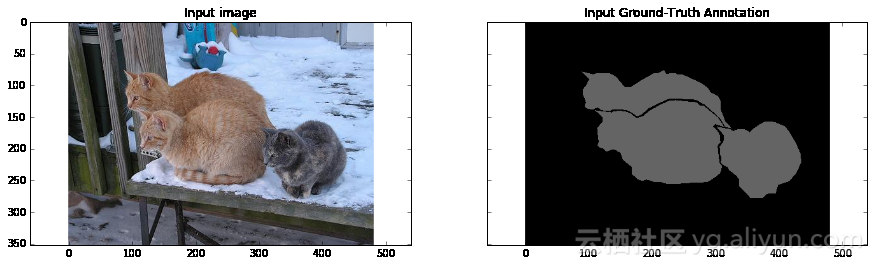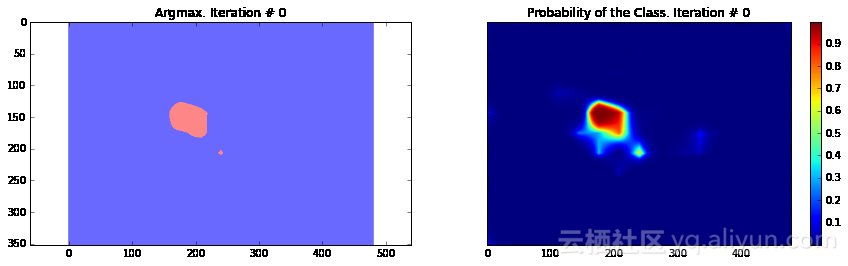Current Loss: 201433.0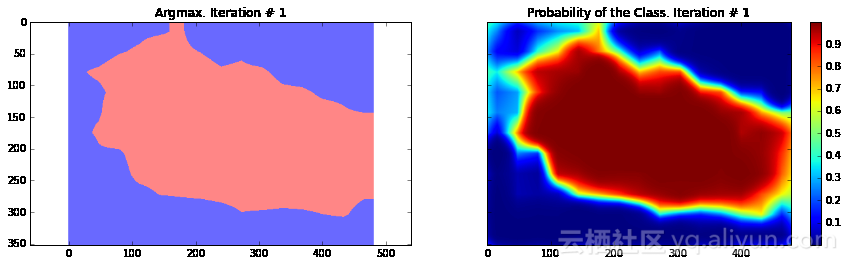Current Loss: 245565.0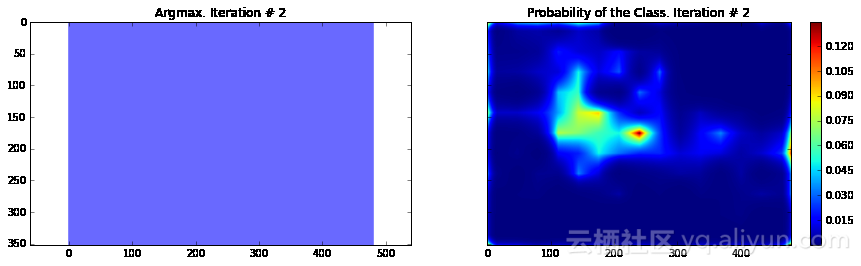Current Loss: 135906.0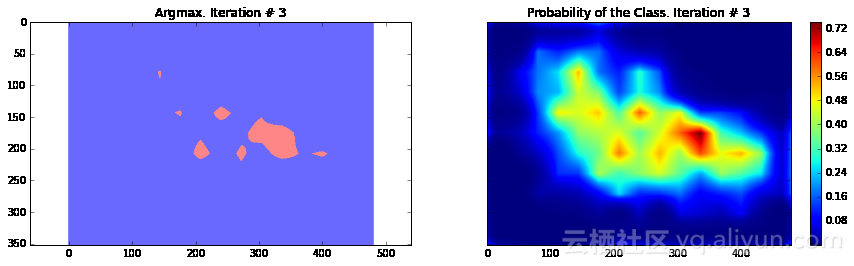Current Loss: 183353.0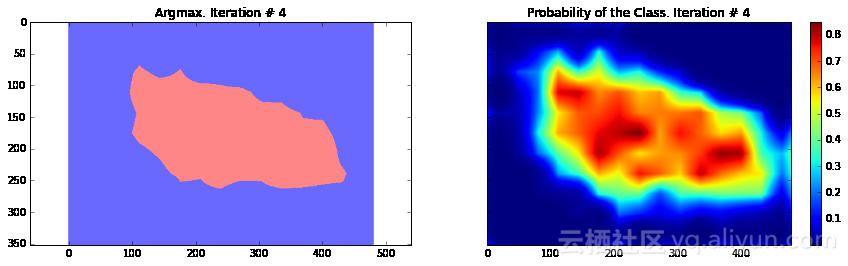Current Loss: 48563.9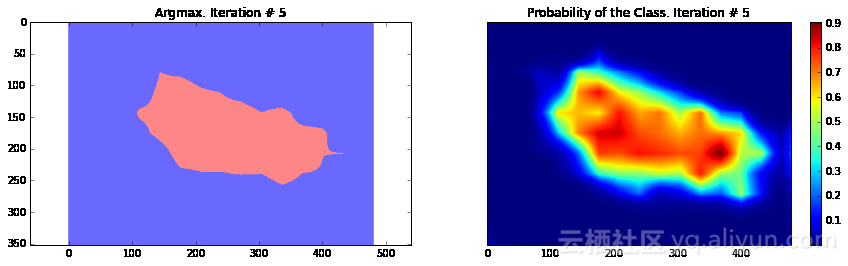Current Loss: 37925.8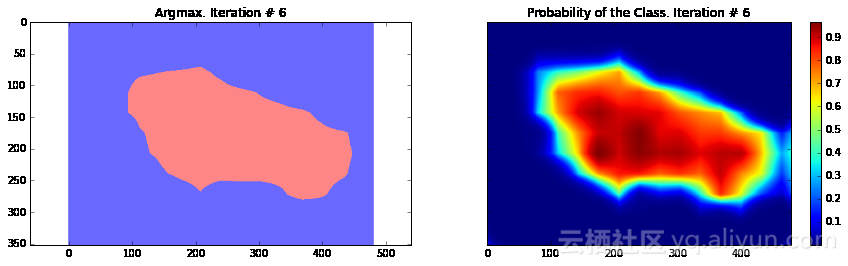Current Loss: 33199.1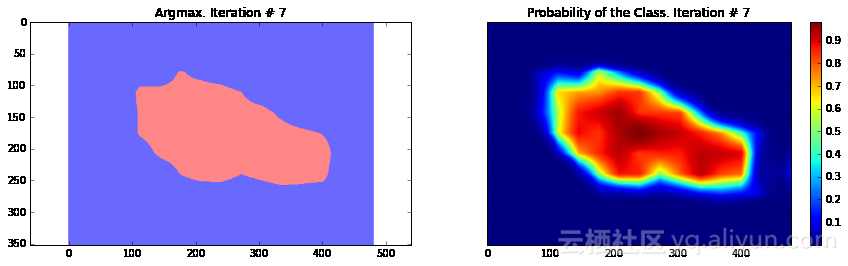Current Loss: 26540.3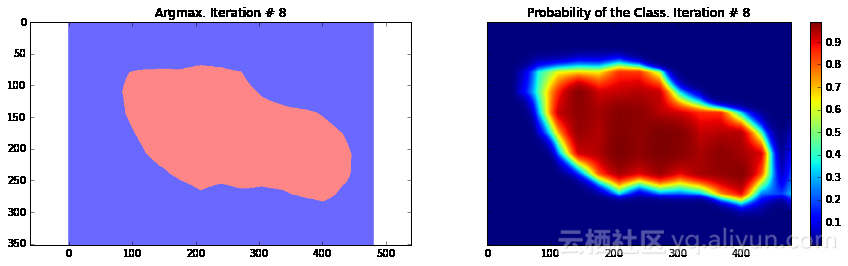Current Loss: 23658.0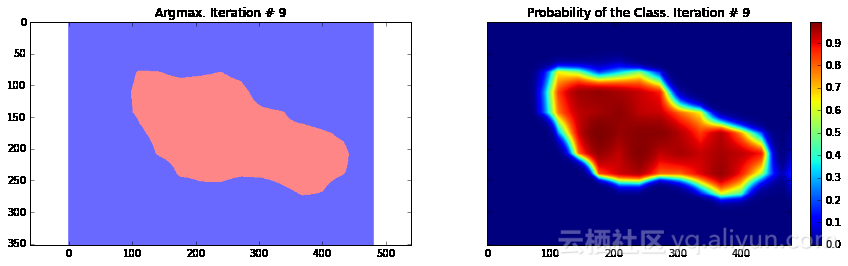Current Loss: 29404.9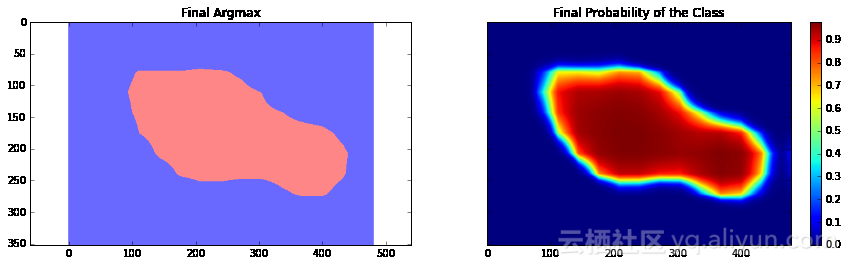Final Loss: 18177.5

条件随机场后处理

import sys

path = "/home/dpakhom1/dense_crf_python/"
sys.path.append(path)

import pydensecrf.densecrf as dcrf

from pydensecrf.utils import compute_unary, create_pairwise_bilateral, \
create_pairwise_gaussian, softmax_to_unary

import skimage.io as io

image = train_image

softmax = final_probabilities.squeeze()

softmax = processed_probabilities.transpose((2, 0, 1))

# 输入数据应为概率值的负对数
# 你可以在softmax_to_unary函数的定义中找到更多信息
unary = softmax_to_unary(processed_probabilities)

# 输入数据应为C-连续的——我们使用了Cython封装器
unary = np.ascontiguousarray(unary)

d = dcrf.DenseCRF(image.shape * image.shape, 2)

d.setUnaryEnergy(unary)

# 潜在地对空间上相邻的小块分割区域进行惩罚——促使产生更多空间连续的分割区域
feats = create_pairwise_gaussian(sdims=(10, 10), shape=image.shape[:2])

kernel=dcrf.DIAG_KERNEL,
normalization=dcrf.NORMALIZE_SYMMETRIC)

# 这将创建与颜色相关的图像特征——因为我们从卷积神经网络中得到的分割结果非常粗糙，
# 我们可以使用局部的颜色特征来改善分割结果
feats = create_pairwise_bilateral(sdims=(50, 50), schan=(20, 20, 20),
img=image, chdim=2)

kernel=dcrf.DIAG_KERNEL,
normalization=dcrf.NORMALIZE_SYMMETRIC)
Q = d.inference(5)

res = np.argmax(Q, axis=0).reshape((image.shape, image.shape))

cmap = plt.get_cmap('bwr')

f, (ax1, ax2) = plt.subplots(1, 2, sharey=True)
ax1.imshow(res, vmax=1.5, vmin=-0.4, cmap=cmap)
ax1.set_title('Segmentation with CRF post-processing')
probability_graph = ax2.imshow(np.dstack((train_annotation,)*3)*100)
ax2.set_title('Ground-Truth Annotation')
plt.show()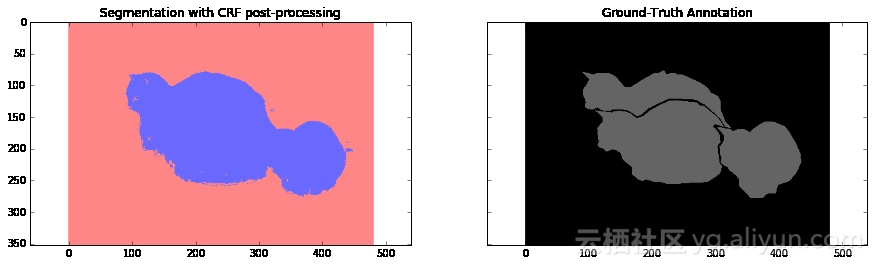结论与讨论

以下为译文

**本文由北邮@爱可可-爱生活 老师推荐，阿里云云栖社区组织翻译。IBM Watson AI：这些公司正在用认知计算打击网络犯罪
1106 0962 0CNN基础知识介绍及TensorFlow具体实现，对于初学者或者求职者而言是一份不可多得的资料。
16169 04469 0Android开发18——获取网络资源之json数据

1067 0827 020765 02940 0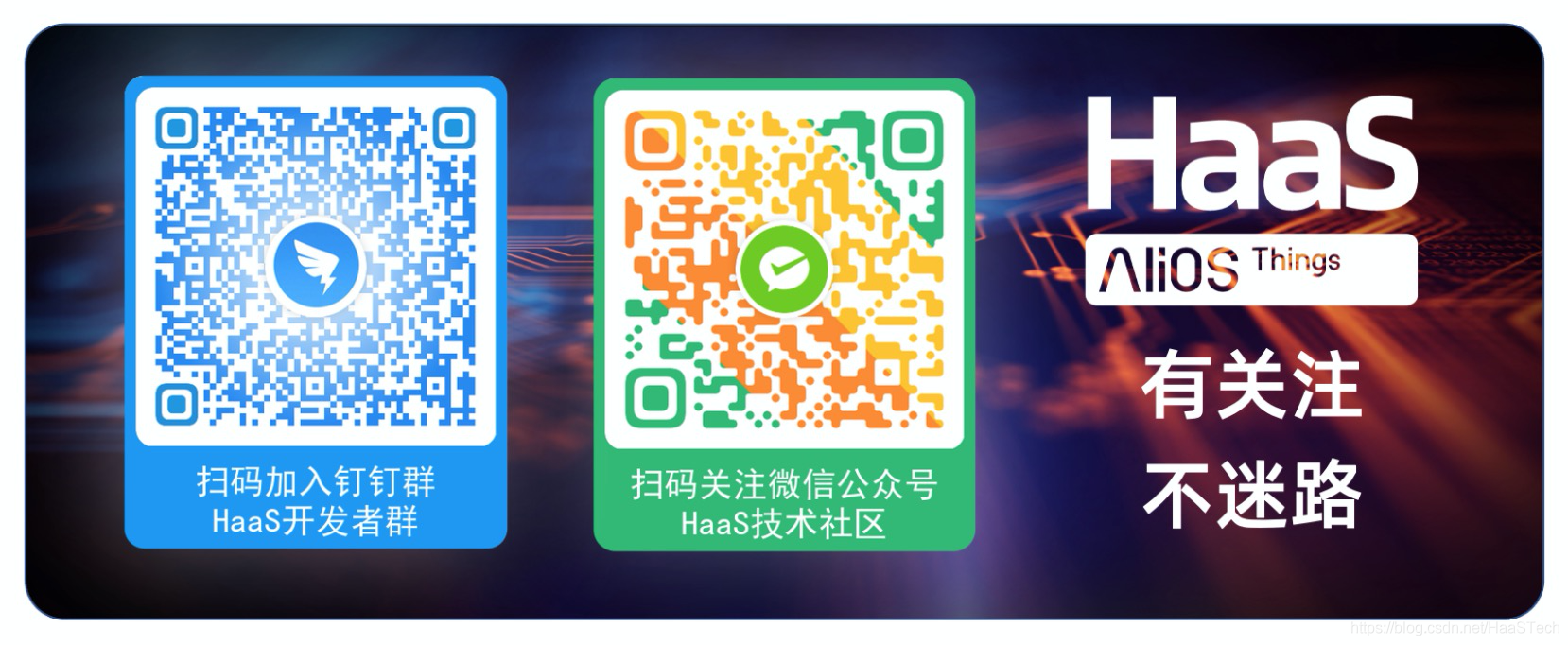JavaScript轻应用网络模块介绍
JavaScript轻应用提供了网络管理相关的功能，APP可以通过JSAPI获取网络相关的信息，并且可以接收网络连接、断开的事件。
49 0【沉淀】从网络中间件到搜索，从移动开发到分布式计算平台，阿里高级专家李睿博谈自己的折腾路

25794 0
【方向】

707

5

《2021云上架构与运维峰会演讲合集》

《零基础CSS入门教程》

《零基础HTML入门教程》